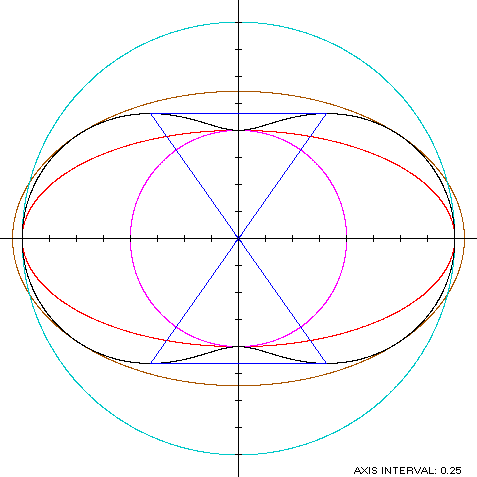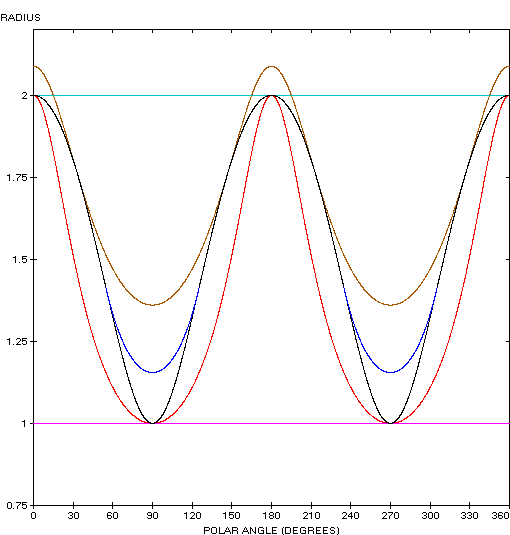Hippopede
HIPPOPEDE

Balmoral Software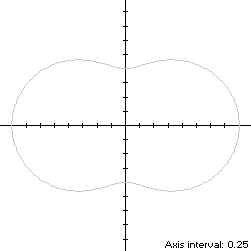The hippopede, also known as the Lemniscate of Booth, is a peanut-shaped bisymmetric curve S with polar equation
r2(t) = 4 - 3sin2(t), 0 ≤ t < 2π
The curve is traced out in a counterclockwise direction, starting from its right edge at (2,0). Its abscissa extrema are (±2,0) and its ordinate extrema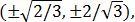so the hippopede is non-convex by the multiple local extrema test. In the first quadrant, the ordinate maximum is achieved when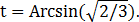The width x height of its bounding rectangle is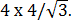### Metrics

We have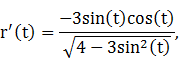so by (L2), the perimeter of S is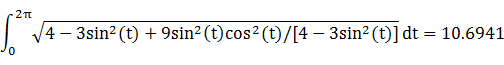and by (A2), the area of S is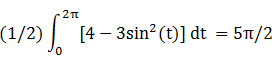### Convex Hull

The convex hull is created by connecting the extreme ordinate points with two horizontal line segments of length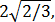so by (L2), the perimeter of the convex hull is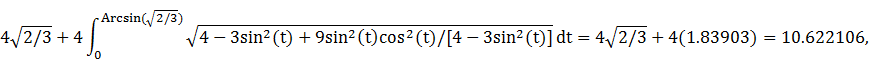which is less than 1% shorter than that of the hippopede.

The line segments of the convex hull create two isosceles triangles with the origin, each having an area of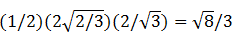as shown in blue in the left diagram below. By (A2), the area of the convex hull is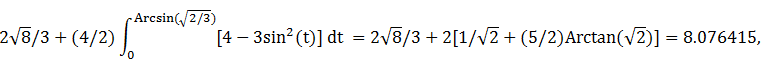which is about 3% more than the area of the hippopede.

### Boundary Circles & Inellipse

The radius extrema are on the coordinate axes at (0,±1) and (±2,0), so the inradius is 1 and the circumradius is 2. A candidate for the inellipse is one enclosed by the annulus between these two circles, with a = 2 and b = 1. For verification, we have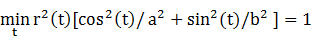### Circumellipse

From Lemma B, we have
x(t)y(t) = [4 - 3sin2(t)]cos(t)sin(t)
This expression is maximized in the first quadrant at the complicated value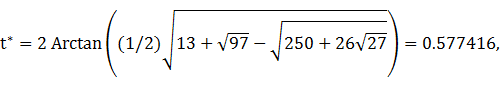which is close to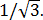The circumellipse dimensions are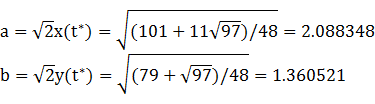For verification, we have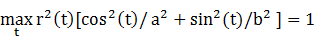### Summary Table

 Perimeter Area Centroid Figure Parameters Incircle R = 1 6.283185 3.141593Inellipse a = 2b = 1 9.688448 6.283185 Hippopede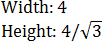10.6941 7.853982 Convex hull 10.622106 8.076415 Circumellipse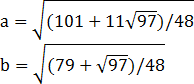10.955915 8.926023 Circumcircle R = 2 12.566371 12.566371